# 一二三年级等量代换的题目怎么做？家长来看看

2021-01-04 90 ℃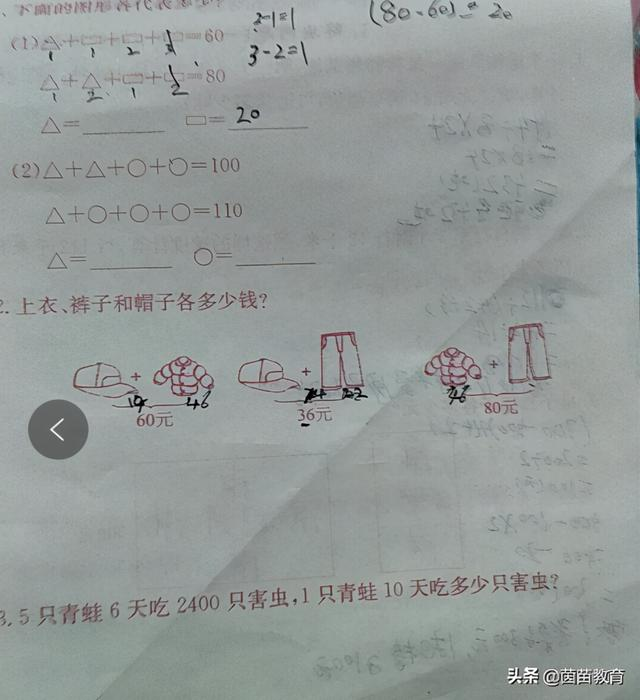1、（1）△+□+□+□=60①

△+△+□+□=80②

①×2得：

△+△+□+□+□+□+□+□=120③

③-②，得

□+□+□+□=40，

△+10+10+10=60，

（2）△+△+○+○=100①

△+○+○+○=110②

②×2，得：

△+△+○+○+○+○+○+○=220③

③-①得

○+○+○+○=120，

△+30+30+30=110，

△=20

2、帽子+上衣=60元①

①+②-③，得：

=60元+36元-80元

3、5只青蛙一天吃的害虫：

2400÷6=400（只）

1只青蛙一天吃的害虫：

400÷5=80（只）

1只青蛙10天吃的害虫：

80×10=800（只）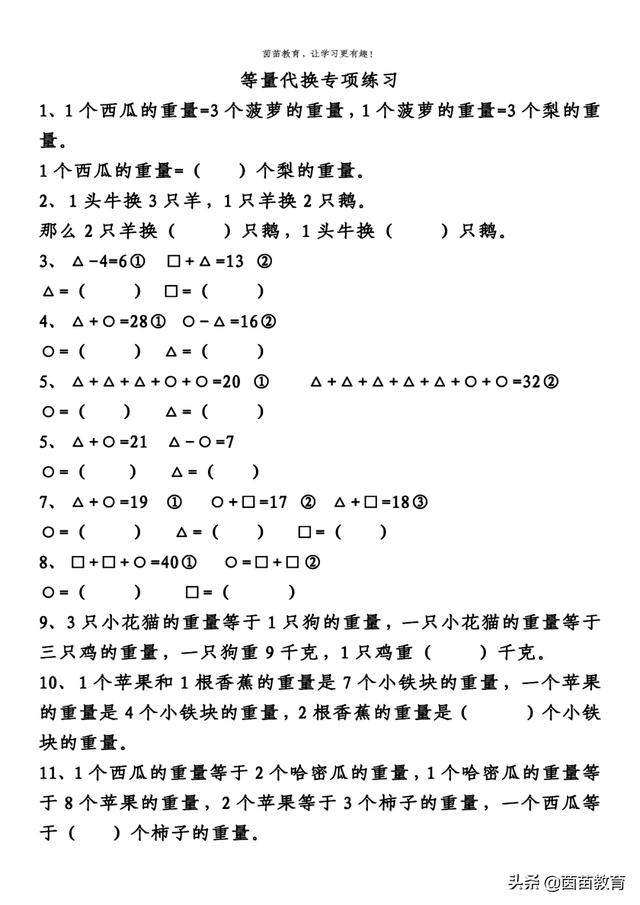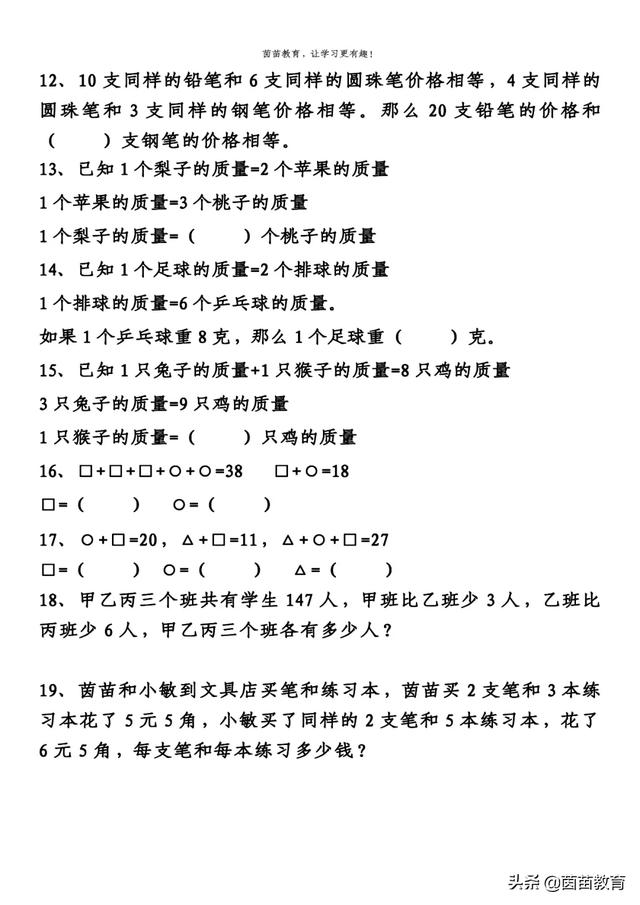1、1个西瓜的重量=3个菠萝的重量，1个菠萝的重量=3个梨的重量。

1个西瓜的重量=（9 ）个梨的重量。

2、1头牛换3只羊，1只羊换2只鹅。

3、△-4=6① □+△=13 ② △=（10）□=（3）

4、△+○=28① ○-△=16② ○=（ 22 ）△=（6 ）

5、△+△+△+○+○=20 ① △+△+△+△+△+○+○=32②

○=（ 1 ）△=（6）

6、△+○=21 △-○=7 ○=（7 ）△=（ 14）

7、△+○=19 ① ○+□=17 ② △+□=18③

○=（9）△=（10）□=（8）

8、□+□+○=40① ○=□+□② ○=（20 ）□=（10）

9、3只小花猫的重量等于1只狗的重量，一只小花猫的重量等于三只鸡的重量，一只狗重9千克，1只鸡重（1）千克。

10、1个苹果和1根香蕉的重量是7个小铁块的重量，一个苹果的重量是4个小铁块的重量，2根香蕉的重量是（6）个小铁块的重量。

11、1个西瓜的重量等于2个哈密瓜的重量，1个哈密瓜的重量等于8个苹果的重量，2个苹果等于3个柿子的重量，一个西瓜等于（24）个柿子的重量。

12、10支同样的铅笔和6支同样的圆珠笔价格相等，4支同样的圆珠笔和3支同样的钢笔价格相等。那么20支铅笔的价格和（9）支钢笔的价格相等。

13、已知1个梨子的质量=2个苹果的质量

1个苹果的质量=3个桃子的质量

1个梨子的质量=（6）个桃子的质量

14、已知1个足球的质量=2个排球的质量

1个排球的质量=6个乒乓球的质量。

8×（6×2）=8×12=96（克）

15、已知1只兔子的质量+1只猴子的质量=8只鸡的质量

3只兔子的质量=9只鸡的质量

1只猴子的质量=（5）只鸡的质量

8-（9÷3）=8-3=5（只）

16、□+□+□+○+○=38 □+○=18

□=（2） ○=（16）

□=38-18×2＝2

○＝18-2＝16

17、○+□=20，△+□=11，△+○+□=27

□=（4） ○=（7） △=（16）

□=○+□+△+□-（△+○+□）=20+11-27=4

18、甲乙丙三个班共有学生147人，甲班比乙班少3人，乙班比丙班少6人，甲乙丙三个班各有多少人？

3乙+3＝147

19、茵苗和小敏到文具店买笔和练习本，茵苗买2支笔和3本练习本花了5元5角，小敏买了同样的2支笔和5本练习本，花了6元5角，每支笔和每本练习多少钱？

2笔+3本=5元5角

2笔+5本=6元5角

6元5角-5元5角=1元=10角

4÷2=2（元）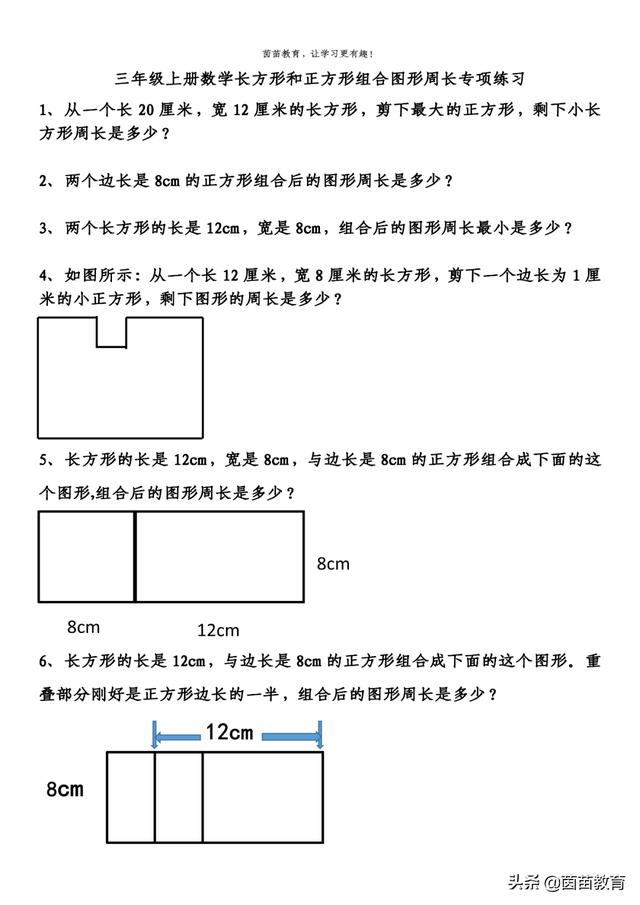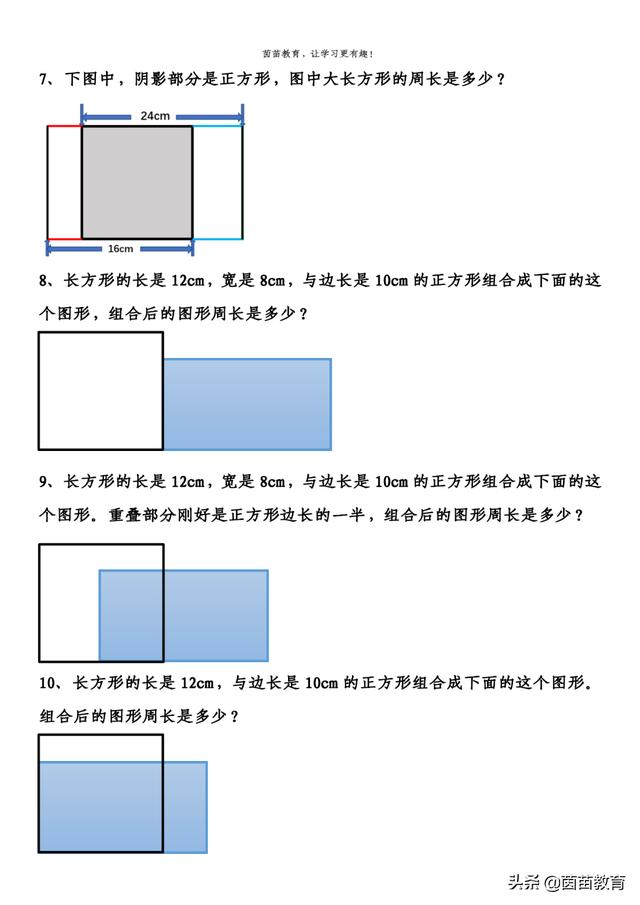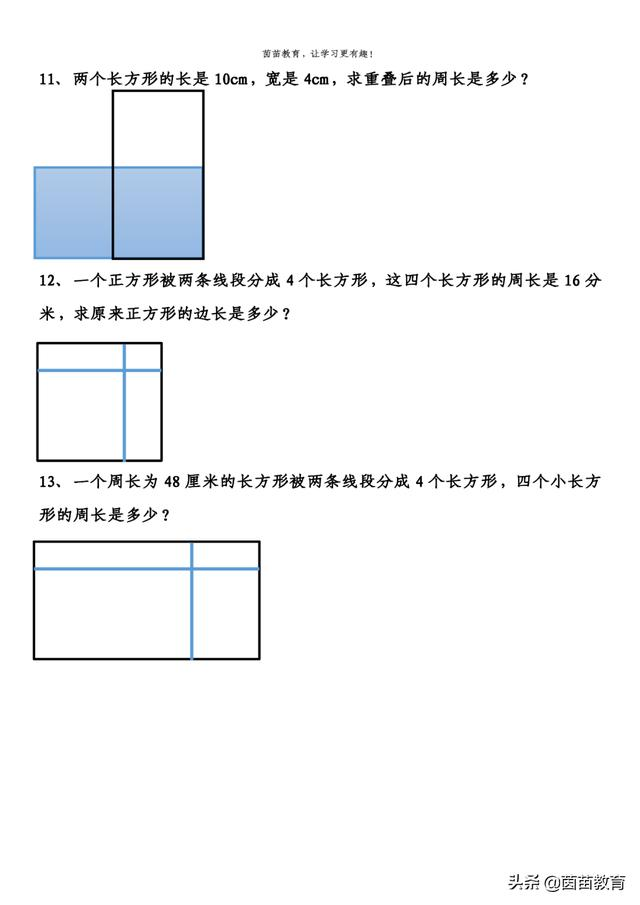1、剩下的小长方形，长为12，宽为20-12=8

（12+8）×2=40（厘米）

2、组合后是一个长方形，长为16cm，宽为8cm

（16+8）=48（cm）

3、把两个长方形的长组合在一起，组成的图形是一个长方形，长为16cm,宽为12cm

（16+12）×2=56（cm）

4、(12+8)×2+2×1=42（厘米）

5、（12+8+8）×2=56（cm）

6、（12+8÷2+8）×2=48（cm）

7、（24+16）×2=80（cm）

8、(10+12+10)×2=64（cm）

9、(10+12-10÷2+10)×2=54（cm）

10、(12+10)×2=44（cm）

11、10×4=40（cm）

12、16÷2÷4=2（分米）

13、48×2=96（厘米）

（此处已添加圈子卡片，请到今日头条客户端查看）